## Print Gallery by M. C. Escher

Chapman研究了Escher的画，Print Gallery。• 在图像中旋转一周，则似乎画面中所有的东西都在放大，但是放大一圈之后，又跟原来一样了。## 山寨一个Escher的画作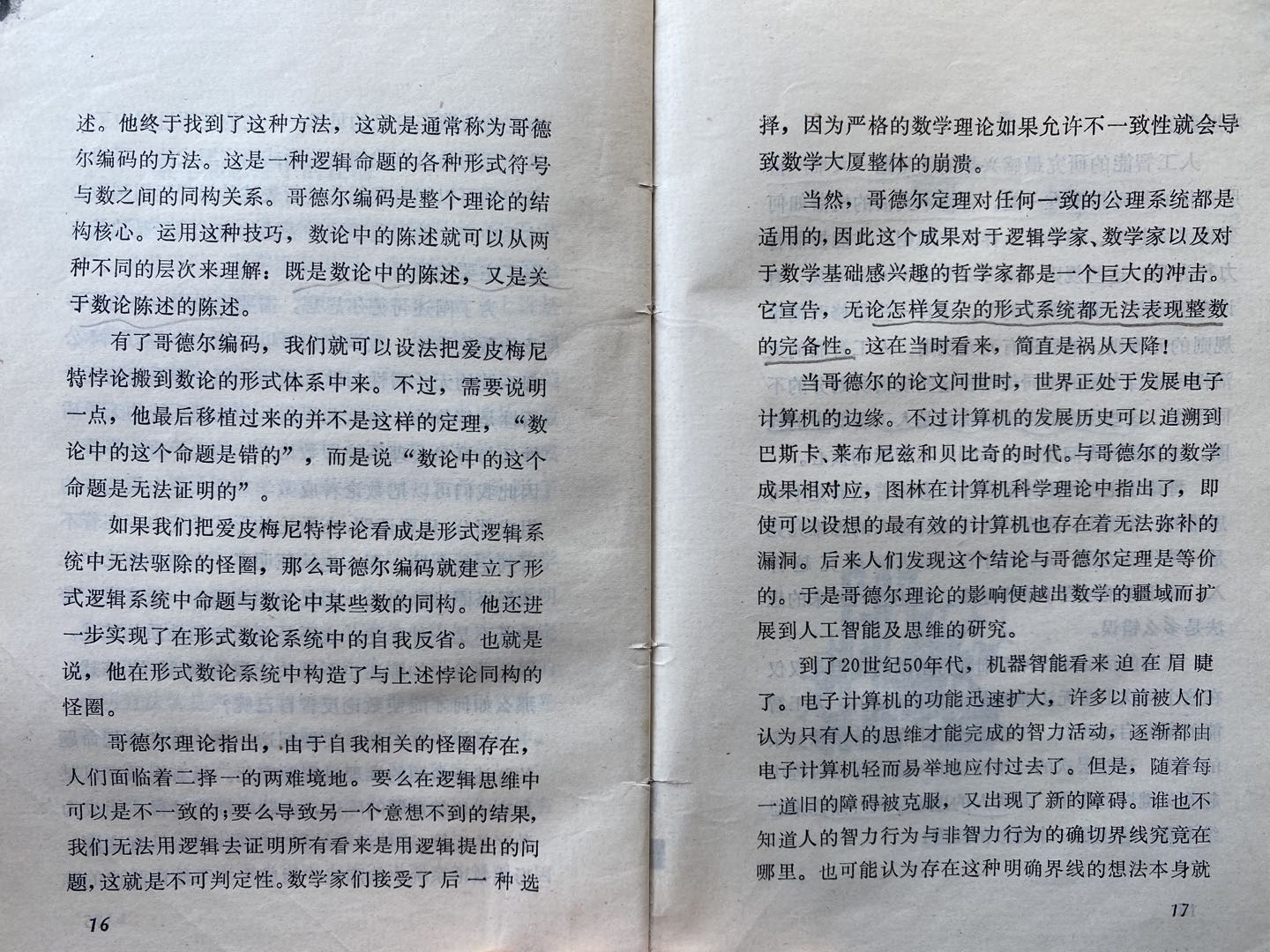## The sum of all natural numbers…

The notion of 1+2+3+4+…=-1/12 has become so wide spread that quite a few of my friends actually feel convinced that it’s actually a true statement in general, even though it is very counter intuitive.

The point is, in order to evaluate an expression, that expression has to be well-defined.

Expressions like a+b is well defined in the sense that we know it is a binary operation, we know what are we supposed to do to get the result.

Expressions like a+b+c+… is not well defined, because there are multiple operators involved, and the order of evaluation is not clearly specified.

Take the sum of the alternating series as an example:

《形而上学》亚里士多德

# 2.1 埃及乘法

$$1a=a$$                                   （2.1）

$$(n+1)a=na+a$$                 （2.2）

int multiply0(int n, int a){
if (n==1) return a;
return multiply0(n-1, a)+a;
}

Ahmes所描述的算法，这个算法在古希腊被称为“埃及乘法”，而很多现代作者则称之为“俄罗斯农民算法”，建立在如下洞察上：

$$4a=((a+a)+a)+a=(a+a)+(a+a)$$

$$a+(b+c)=(a+b)+c$$

1        ♦         59
2                  118
4                  236
8        ♦        472
16                944
32      ♦      1888

$$41*59=(1*59)+(8*59)+(32*59)$$

$$n=n/2+n/2$$    说明n是偶数
$$n=(n-1)/2+(n-1)/2+1$$  说明n是奇数

odd(n) 意味着 half(n)=half(n-1)

int multiply1(int n, int a){
if (n==1) return a;
int result=multiply1(half(n), a+a);
if (odd(n)) result=result +a;
return result;
}

odd(x)很容易实现，测试x的最低位就可以了，half(x)则可以通过对x进行一次右移实现：

bool odd(int n) { return n&0x1;}
int half(int n) { return n>>1; }

multiply1需要进行多少次加法运算？每次调用这个函数，我们需要在a+a的地方进行一次加法，因为我们是不断对n取半，我们需要调用这个函数logn次。在某些时候，我们还需要在result+a的地方进行加法运算，因此加法运算的总次数为：

$$\#(n)=logn + v(n)-1$$

$$\#(15)=3+4-1=6$$

int multiply_by_15(int a){
int b=(a+a)+a;    //b == 3*a
int c+b+b;        //c == 6*a
return (c+c)+b;   //12*a + 3*a
}

# 2.2 改进算法

r+na

int mult_acc0{int r, int n, int a) {
if (n==1) return r+a;
if (odd(n)){
return mult_acc0(r+a, half(n), a+a);
}else{
return mult_acc0(r, half(n), a+a);
}
}

int mult_acc1(int r, int n, int a) {
if (n==1) return r+a;
if (odd(n)) r=r+a;
return mult_acc1(r, half(n), a+a);
}

• n=1的情况很少发生；
• 如果n是偶数，则完全没有必要判断它还是不是1.

int mult_acc2(int r, int n, int a) {
if (odd(n)) {
r=r+a;
if (n==1) return r;
}
return mult_acc2(r, half(n), a+a);
}

int mult_acc3 (int r, int n, int a) {
if (odd(n)) {
r=r+a;
if (n==1) return r;
}
n=half(n);
a=a+a;
return mult_acc3(r,n,a);
}

int mult_acc4(int r, int n, int a) {
while (true) {
if (odd(n)) {
r=r+a;
if (n==1) return r;
}
n=half(n);
a=a+a;
}
}

int multiply2(int n, int a) {
if (n==1) return a;
return mult_acc4(a, n-1, a);
}

int multiply3(int n, int a) {
while (!odd(n)) {
a=a+a;
n=half(n);
}
if (n==1) return a;
return mult_acc4(a, n-1, a);
}

int multiply4(int n, int a) {
while (!odd(n)) {
a=a+a;
n=half(n);
}
if (n==1) return n;
return mult_acc4(a, half(n-1), a+a);
}

# 2.3 本章的思考

## a new way of defining $$e$$ the Euler number

This is inspired by a post on Quora by Alon Amit. I found this approach of defining e, the Euler number more intuitive than the traditional way($$e=\lim_{n \to \infty} (1+\frac{1}{n})^n$$). In addition, it explains the importance of the Euler number very well.

First of all, we need a function that solves the elementary differential equation:

$$\frac{d f(x)}{d x} = f(x)$$

Of course, without specifying an initial value, this equation will have infinite number of solutions. Just for convenience, let’s specify:

$$f(0)=1$$

By Picard–Lindelöf theorem, our equation:

$$\begin{gather*} \frac{d f(x)}{d x} = f(x)\\f(0)=1 \end{gather*}$$

has one and only one solution.

Now, let’s see what kind of property this function $$f(x)$$ has.

## 另外一种定义欧拉数$$e$$的方法

$$e=\lim_{n \to \infty} (1+\frac{1}{n})^n$$

$$f’=f$$                         ……（1）

$$f(x+a)=c*f(x)$$                     ……（2）

$$f(x+a)=f(a)*f(x)$$

$$f(1)=exp(1)=1+1+\frac{1}{2!}+\frac{1}{3!}+…+\frac{1}{n!}+…$$

$$\begin{gather*} \frac{d f(x)}{d x} = f(x)\\f(0)=1 \end{gather*}$$

$$\frac{d^2I}{dt^2}=-cI$$，其中$$c$$为大于零的常数。

$$k^2*e^{k*t}=-c*e^{k*t}$$，因此，

$$k^2=-c\\k=\pm\sqrt{c}*i$$

The reason this number e is considered the “natural” base of exponential functions is that this function is its own derivative.

This natural exponential function is identical with its derivative. This is really the source of all the properties of the exponential function, and the basic reason for its importance in applications…

## 音高不等于频率https://en.wikipedia.org/wiki/Shepard_tone

## composing using Matlab – part 1

I have been attempted to do this for a long time, but only now I have some time to actually try it out. As it turned out, to have Matlab playing music is relatively easy, to have Matlab playing appealing music is way harder than I have imagined.

The core part is this Matlab function:

sound(v);

This function takes a vector and simply output it to the audio device in sequence. Values in the vector is the relative amplitude. Values larger than 1 will be ignored.

The default sample rate is 8000, so you’ll need 8000 data point to get 1 second of sound. If we want higher sample rate, then have to play the vector at a higher sample rate:

sound(v,16000);

Now, it’s very easy to generate such a vector:

First, generate a vector that represents time points:

tp=0:1/16000:0.5;
v=sin(440*2*pi*tp);

The first line generates a vector of 0.5*16000 values. That is 0.5 seconds in 16000 sample rate. The second line generates a sine wave of 440Hz for 0.5 seconds.

440Hz is A4. 12 half tones double the frequency. From C4 to A4 is 9 half tones. So C4 is 440*2^(-9/12)Hz. So for a simple C4 to C5, here’s the frequencies:

σ=2^(1/12)

 Note Numbered Notation Frequency C 1 440*σ^-9 C# 440*σ^-8 D 2 440*σ^-7 D# 440*σ^-6 E 3 440*σ^-5 F 4 440*σ^-4 F# 440*σ^-3 B 5 440*σ^-2 B# 440*σ^-1 A 6 440 A# 440*σ G 7 440*σ^2 C i 440*σ^3

So we can simply do this:

n1=sin(440*2^(-9/12)*2*pi*tp);         % C
n2=sin(440*2^(-9/12)*2*pi*tp);         % C
n3=sin(440*2^(-2/12)*2*pi*tp);         % B
n4=sin(440*2^(-2/12)*2*pi*tp);         % B
n5=sin(440*2^(0/12)*2*pi*tp);          % A
n6=sin(440*2^(0/12)*2*pi*tp);          % A
n7=sin(440*2^(-2/12)*2*pi*tp);         % B
sound(n1,16000);
sound(n2,16000);
sound(n3,16000);
sound(n4,16000);
sound(n5,16000);
sound(n6,16000);
sound(n7,16000);

This gives us “twinkle twinkle little star”. Here I output the audio into .wav file:

wavwrite([n1,n2,n3,n4,n5,n6,n7],16000,'c:\twinkle.wav');

Easy, right?

On hearing it, you notice that, it sounds very uncomfortable, and there’s no variation of amplitude within one note.

The problem is, I’m using “pure tone” here. Real instruments generate sound with a very rich composition of different frequency, phase and amplitude, that we haven’t take into consideration here. Next post I’ll try to mimic the sound generated by a hitting a key on a piano.

## Linear regression in Excel – continued

Doing linear regression in Excel is simple. Now I’d like to show you how exactly this is done.

Let’s use the same data set:

 x 9.42 2.78 5.87 5.49 6.48 4.91 7.82 2.7 2.65 8.42 5.85 0.93 y 52.24 4.15 13.72 7 5.28 6.98 42 7.15 3.41 31 12.59 1.09

Let’s say we want do a order-2 linear regression. That means, we want a function:

f(x)=c*x^2 + b*x+a

a, b and c should be chosen in a way such that if we put x into f(x), the difference/error between f(x) and y, will be minimized, in the least square sense.

So we have the difference R as a function of a,b,c：

R(a,b,c)=(c* 9.42^2 + b* 9.42 + a – 52.24)^2+(c*2.78^2+b*2.78+a-4.15)^2+…+(c*0.93^2+b*0.93+a)

R is smooth every where in space. So R is minimal where:

D(R)/D(a)=0; D(R)/D(b)=0; D(R)/D(c)=0

Now we’ll have to employ the compact form to avoid tedious typing: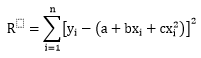In order for R to be minimal, we need: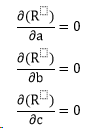Insert R into the equations and expand them, we get: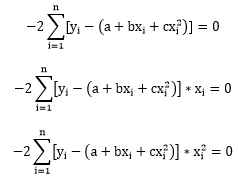That is: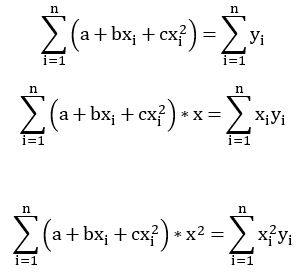Or in matrix form: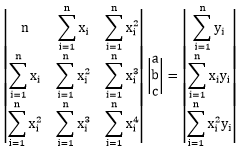Now this is a very simple equation, so we can simply solve it using Cramer’s rule:It looks scary but actually not too complex. We need the sum of x, x^2, x^3, x^4, x*y and x^y. We can actually verify this in excel:

 x y x^2 x^3 x^4 x*y x^2*y 9.42 52.24 88.69578 835.323 7866.942 491.974 4633.334 2.78 4.15 7.721688 21.45697 59.62447 11.53932 32.06537 5.87 13.72 34.43254 202.0476 1185.6 80.52797 472.5321 5.49 7.00 30.18894 165.8715 911.3719 38.47401 211.3934 6.48 5.28 41.99053 272.099 1763.204 34.19838 221.6059 4.91 6.98 24.08858 118.227 580.2595 34.23907 168.0457 7.82 42.00 61.22442 479.0568 3748.43 328.6334 2571.426 2.70 7.15 7.269068 19.59829 52.83935 19.27266 51.96143 2.65 3.41 7.003793 18.53532 49.05312 9.028243 23.89296 8.42 31.00 70.90802 597.0945 5027.948 261.0414 2198.149 5.85 12.59 34.27254 200.6409 1174.607 73.71933 431.5732 0.93 1.09 0.869064 0.810173 0.755272 1.014426 0.945685 63.32 186.61 408.665 2930.761 22420.63 1383.662 11016.92

So we have: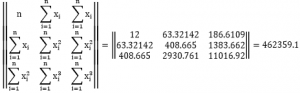So in the end we get: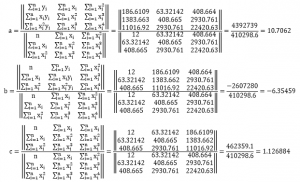This is exactly what Excel displayed on the chart: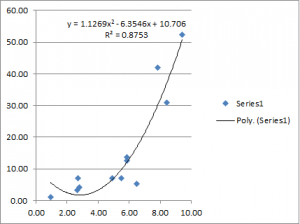I actually put all this in an Excel file so you can try it yourself. Please note that in the excel file we have a data set of 12 points. If your data set has a different size, you may have to adapt the formulas a little bit. 🙂

## Linear regression in Excel

Excel has a very handy feature that does linear regression for you very intuitively.

Let’s say you have a data set like this:

 x 9.42 2.78 5.87 5.49 6.48 4.91 7.82 2.7 2.65 8.42 5.85 0.93 y 52.24 4.15 13.72 7 5.28 6.98 42 7.15 3.41 31 12.59 1.09

First, you’ll have to insert a scatter chart:You’ll get a chart like this (Excel is smart enough to figure out what should be the x-axis and what should be the y-axis):Now, right click on the dots and then you can select “Add Trendline…” from the pop-up menu.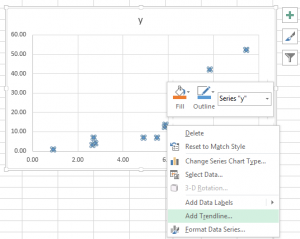Then you’ll be presented with a dialog like this:Note that Excel has different options for “Linear” and “Polynomial”. Here the option is about the relationship between the variables. All these different options are all Linear Regressions.

Let’s try a order 2 polynomial regression and check the option “Display Equation on chart” and “Display R-squared value on chart”. This is what you’ll get:Good thing about doing this in Excel is, you can always change the data set and your chart and equation will be updated automatically.

Depending on the nature of your data set, you may want to try different model to get a better fit, the procedure will be the same.